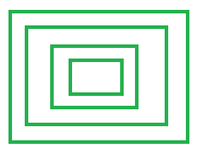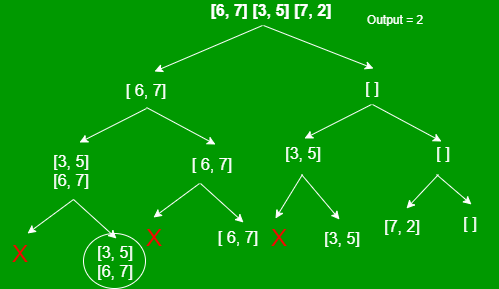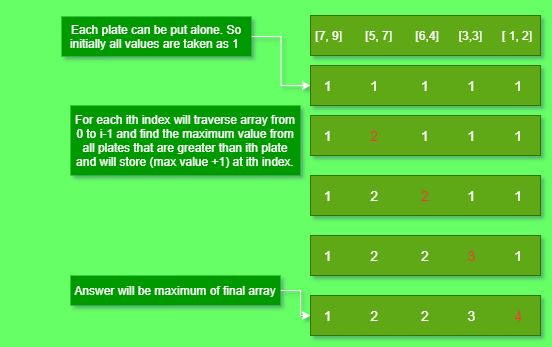Related Articles

# Maximum number of plates that can be placed from top to bottom in increasing order of size

• Last Updated : 16 Jun, 2021

Given a 2D array plates[][] of size N, which each row representing the length and width of a N rectangular plates, the task is to find the maximum number of plates that can be placed on one another.
Note: A plate can be put on another only if its length and width are strictly smaller than that plate.Examples:

Input: Plates[][] = [ [3, 5], [6, 7], [7, 2], [2, 3] ]
Output:
Explanation: Plates can be arranged in this manner [ 6, 7 ] => [ 3, 5 ] => [ 2, 3 ].

Input: Plates[][] = [ [6, 4], [ 5, 7 ], [1, 2], [ 3, 3 ], [ 7, 9 ] ]
Output:
Explanation: Plates can be arranged in this manner [ 7, 9 ] => [ 5, 7 ] => [ 3, 3 ] => [ 1, 2 ].

Approach: The problem is a variation of the Longest increasing subsequence problem. The only difference is that in LIS, if i < j, then ith element will always come before the jth element. But here, choosing of plates doesn’t depend on index. So, to get this index restriction, sorting all the plates in decreasing order of area is required.

If (i < j) and area of ith plate is also greater than jth plate, then ith plate will always come before(down) the jth plate.

#### Recursive approach:

Two possible choices exists for each plate, i.e. either to include it in the sequence or discard it. A plate can be included only when its length and width are smaller than the previous included plate.

Recursion tree for the array plates[][] = [ [6, 7], [3, 5], [7, 2] ] is as follows:Below is the implementation of the recursive approach:

## C++

 `// C++ Program for the above approach` `#include ``using` `namespace` `std;` `// Comparator function to sort plates``// in decreasing order of area``bool` `comp(vector<``int``> v1,``          ``vector<``int``> v2)``{``    ``return` `v1 * v1 > v2 * v2;``}` `// Recursive function to count and return``// the max number of plates that can be placed``int` `countPlates(vector >& plates,``                ``int` `lastLength, ``int` `lastWidth,``                ``int` `i, ``int` `n)``{``    ``// If no plate remains``    ``if` `(i == n)``        ``return` `0;` `    ``int` `taken = 0, notTaken = 0;` `    ``// If length and width of previous plate``    ``// exceeds that of the current plate``    ``if` `(lastLength > plates[i]``        ``&& lastWidth > plates[i]) {` `        ``// Calculate including the plate``        ``taken = 1 + countPlates(plates, plates[i],``                                ``plates[i], i + 1, n);` `        ``// Calculate excluding the plate``        ``notTaken = countPlates(plates, lastLength,``                               ``lastWidth, i + 1, n);``    ``}` `    ``// Otherwise``    ``else` `        ``// Calculate only excluding the plate``        ``notTaken = countPlates(plates, lastLength,``                               ``lastWidth, i + 1, n);` `    ``return` `max(taken, notTaken);``}` `// Driver code``int` `main()``{``    ``vector > plates = { { 6, 4 }, { 5, 7 },``                        ``{ 1, 2 }, { 3, 3 }, { 7, 9 } };``    ``int` `n = plates.size();` `    ``// Sorting plates in decreasing order of area``    ``sort(plates.begin(), plates.end(), comp);` `    ``// Assuming first plate to be of maximum size``    ``int` `lastLength = INT_MAX;``    ``int` `lastWidth = INT_MAX;` `    ``cout << countPlates(plates, lastLength,``                        ``lastWidth, 0, n);``    ``return` `0;``}`

## Java

 `// Java program for the above approach``import` `java.lang.*;``import` `java.util.*;` `class` `GFG{` `// Recursive function to count and return``// the max number of plates that can be placed``static` `int` `countPlates(``int``[][] plates,``                       ``int` `lastLength,``                       ``int` `lastWidth,``                       ``int` `i, ``int` `n)``{``    ` `    ``// If no plate remains``    ``if` `(i == n)``        ``return` `0``;` `    ``int` `taken = ``0``, notTaken = ``0``;` `    ``// If length and width of previous plate``    ``// exceeds that of the current plate``    ``if` `(lastLength > plates[i][``0``] &&``        ``lastWidth > plates[i][``1``])``    ``{``        ` `        ``// Calculate including the plate``        ``taken = ``1` `+ countPlates(plates, plates[i][``0``],``                                ``plates[i][``1``], i + ``1``, n);` `        ``// Calculate excluding the plate``        ``notTaken = countPlates(plates, lastLength,``                               ``lastWidth, i + ``1``, n);``    ``}` `    ``// Otherwise``    ``else``    ` `        ``// Calculate only excluding the plate``        ``notTaken = countPlates(plates, lastLength,``                               ``lastWidth, i + ``1``, n);` `    ``return` `Math.max(taken, notTaken);``}` `// Driver code``public` `static` `void` `main(String[] args)``{``    ``int``[][] plates = { { ``6``, ``4` `}, { ``5``, ``7` `},``                       ``{ ``1``, ``2` `}, { ``3``, ``3` `}, { ``7``, ``9` `} };``    ``int` `n = plates.length;``    ` `    ``// Sorting plates in decreasing order of area``    ``Arrays.sort(plates, (v1, v2)-> (v2[``0``] * v2[``1``]) -``                                   ``(v1[``0``] * v1[``1``]));``    ` `    ``// Assuming first plate to be of maximum size``    ``int` `lastLength = Integer.MAX_VALUE;``    ``int` `lastWidth = Integer.MAX_VALUE;``    ` `    ``System.out.println(countPlates(plates, lastLength,``                                   ``lastWidth, ``0``, n));``}``}` `// This code is contributed by offbeat`

## Javascript

 ``
Output:
`4`

Time Complexity: O(2N
Auxiliary Space: O(N)

Dynamic Programming Approach: The above approach can be optimized using Dynamic programming as illustrated below.Below is the implementation of the above approach:

## C++

 `// C++ Program for the above approach` `#include ``using` `namespace` `std;` `// Comparator function to sort plates``// in decreasing order of area``bool` `comp(vector<``int``> v1, vector<``int``> v2)``{``    ``return` `v1 * v1 > v2 * v2;``}` `// Function to count and return the max``// number of plates that can be placed``int` `countPlates(vector >& plates, ``int` `n)``{` `    ``// Stores the maximum``    ``// number of plates``    ``int` `maximum_plates = 1;``    ``vector<``int``> dp(n, 1);` `    ``for` `(``int` `i = 1; i < n; i++) {``        ``int` `cur = dp[i];` `        ``// For each i-th plate, traverse``        ``// all the previous plates``        ``for` `(``int` `j = i - 1; j >= 0; j--) {` `            ``// If i-th plate is smaller than j-th plate``            ``if` `(plates[i] < plates[j]``                ``&& plates[i] < plates[j]) {` `                ``// Include the j-th plate only if current``                ``// count exceeds the previously stored count``                ``if` `(cur + dp[j] > dp[i]) {` `                    ``dp[i] = cur + dp[j];` `                    ``// Update the maximum count``                    ``maximum_plates = max(maximum_plates, dp[i]);``                ``}``            ``}``        ``}``    ``}``    ``return` `maximum_plates;``}` `// Driver code``int` `main()``{``    ``vector > plates = { { 6, 4 }, { 5, 7 },``                        ``{ 1, 2 }, { 3, 3 }, { 7, 9 } };``    ``int` `n = plates.size();` `    ``// Sorting plates in decreasing order of area``    ``sort(plates.begin(), plates.end(), comp);` `    ``cout << countPlates(plates, n);` `    ``return` `0;``}`

## Javascript

 ``
Output:
`4`

Time Complexity: O(N2
Auxiliary Space: O(N)

Attention reader! Don’t stop learning now. Get hold of all the important DSA concepts with the DSA Self Paced Course at a student-friendly price and become industry ready.  To complete your preparation from learning a language to DS Algo and many more,  please refer Complete Interview Preparation Course.

In case you wish to attend live classes with experts, please refer DSA Live Classes for Working Professionals and Competitive Programming Live for Students.

My Personal Notes arrow_drop_up